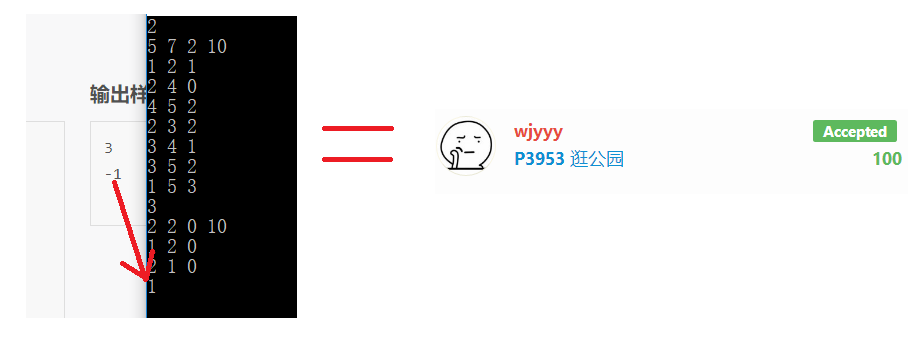# 洛谷 P3953 NOIp2017提高组 逛公园 题解【记忆化搜索】【最短路】

我要是考场上会什么最短路……

## 【输入输出样例 1】

park.in
2
5 7 2 10
1 2 1
2 4 0
4 5 2
2 3 2
3 4 1
3 5 2
1 5 3
2 2 0 10
1 2 0
2 1 0

park.out
3

-1

1–5, 1–2–4–5,1–2–3–5为3条合法路径。

## 前言：

简析一下数据梯度。

10分：floyd求最短路计数。

30分：spfa求最短路计数。

70分：不用判0环（输出-1）的正解。

100分：正解/没有判过1的0环（样例2没过）$\Rightarrow$## 题解：

可以用记忆化搜索过掉，首先SPFA预处理出从1出发的单源最短路径，接着判断有无过1的0环(出于严谨，虽然没有必要)，有则输出-1。考虑类似背包的转移，也就是对于点$v,d[v]+x,x\in [0,k]$，设存在边$(u,v)=w$，则可以由$t=x-[w-(dis[v]-dis[u])]$转移。当w在最短路上时，就是由同层的$x$转移，如果不在最短路上，就是由不同层的$t$转移。当$t\le 0$时，不合法，就不转移。

因为我们的状态是由前面（靠近源点）状态转移过来的，所以记忆化搜索是倒着做的。在做完SPFA后，将图的方向反过来，就是dfs时用的图。那么一个点如果被搜索过，可以直接调用它的状态；如果一个点没有搜索过，就继续搜索它；如果它还在系统栈里，说明找到了环，此时判断上述$t$是否合法，如果不合法则递归，如果合法就当它没有搜索过。如果出现0环，则两次找到某个点时，利用的$x$是相同的，此时判断找到0环，输出-1。

同时，记忆化搜索是要做$k$遍的，因为做第i次时，只有f[u][1…i]是全部有效/合法的。所以做k次保证了所有转移来的都是合法的，不合法的初始值f[i][j]=-1，f=1，存的是方案数。

## Code：

#include<cstdio>
#include<cstring>
#include<queue>
using std::deque;
struct edge
{
int n,v,nxt;
edge(int n,int v,int nxt)
{
this->n=n;
this->v=v;
this->nxt=nxt;
}
edge()
{
nxt=-1;
}
}e,E;
{
}
{
}
int p;
int f;
bool in;
int dis;
void spfa()
{
memset(dis,0x3f,sizeof(dis));
in=true;
dis=0;
deque<int> q;
q.push_back(1);
while(!q.empty())
{
int k=q.front();
q.pop_front();
in[k]=false;
{
if(dis[E[i].n]>dis[k]+E[i].v)
{
dis[E[i].n]=dis[k]+E[i].v;
if(!in[E[i].n])
{
in[E[i].n]=true;
if(q.empty()||dis[E[i].n]<dis[q.front()])
q.push_front(E[i].n);
else
q.push_back(E[i].n);
}
}
}
}
}

bool used;
int flag=0;
int dfs(int x,int k)
{
int t;
if(f[x][k]==-1)
f[x][k]++;
{
int tmp=0;
t=k-(e[i].v-(dis[x]-dis[e[i].n]));
if(t<0)
continue;
if(!used[e[i].n][t]&&~f[e[i].n][t])
f[x][k]+=f[e[i].n][t];
else
{
if(used[e[i].n][t])
{
flag=1;
return 0;
}
used[e[i].n][t]=true;
f[x][k]+=dfs(e[i].n,t);
if(!tmp)
used[e[i].n][t]=false;
if(flag)
return 0;
}
f[x][k]%=p;
}
return f[x][k];
}
bool Used;
int nega;
void Dfs(int x)//判过1的0环
{
if(E[i].v==0)
{
if(Used[E[i].n])
{
nega=1;
return;
}
Used[E[i].n]=true;
Dfs(E[i].n);
}
}

int main()
{
int T,n,m,k;
int u,v,w;
scanf("%d",&T);
while(T--)
{
memset(Used,0,sizeof(Used));
memset(used,0,sizeof(used));
memset(in,0,sizeof(in));
memset(f,-1,sizeof(f));
scanf("%d%d%d%d",&n,&m,&k,&p);
ecnt=-1;
Ecnt=-1;
for(int i=1;i<=m;++i)
{
scanf("%d%d%d",&u,&v,&w);
}
spfa();
Used=true;
nega=0;
Dfs(1);

if(nega)
{
puts("-1");
continue;
}

f=1;
int ans=0;
for(int i=0;i<=k;++i)
{
memset(used,0,sizeof(used));
used[n][i]=true;
flag=0;
f[n][i]=dfs(n,i)%p;
ans+=f[n][i];//答案是全部d+x x∈[0,k]的所有合法方案
ans%=p;
if(flag)
{
ans=-1;
break;
}
}
printf("%d\n",ans);
}
return 0;
}


### 说点什么Subscribe

/* */